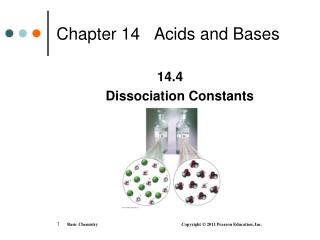DownloadDownload PresentationChapter 14 Acids and Bases

# Chapter 14 Acids and Bases

Télécharger la présentation## Chapter 14 Acids and Bases

- - - - - - - - - - - - - - - - - - - - - - - - - - - E N D - - - - - - - - - - - - - - - - - - - - - - - - - - -
##### Presentation Transcript

1. Chapter 14 Acids and Bases 14.4 Dissociation Constants

2. Strong Acids In water, a strong acid • dissociates into ions • produces high concentrations of H3O+ and an anion A-

3. Weak Acids In a weak acid, • equilibrium favors the undissociated (molecular) form of the acid • the concentrations of H3O+ and anion A- are low HA(aq) + H2O(l) H3O(aq) + A−(aq)

4. Characteristics of Acids and Bases

5. Acid Dissociation Constant A weak acid has • an equation for dissociation written HA(aq) + H2O(l) H3O+(aq) + A(aq) • an equilibrium expression of Keq = [H3O+][A] [HA][H2O] • a Ka expression written without [H2O], which is a liquid and is a constant Ka = [H3O+][A] [HA]

6. Acid and Base Dissociation Constants

7. Writing a Ka for a Weak Acid Write the Ka for H2S. 1. Write the equation for the dissociation of H2S. H2S(aq) + H2O(l) H3O+(aq) + HS−(aq) 2. Set up the Ka expression Ka = [H3O+][HS-] [H2S]

8. Learning Check Write the Ka for the weak acid HCN.

9. Solution HCN (aq)+ H2O(l) H3O+ (aq) + CN− (aq) Set up the Ka expression Ka = [H3O+][CN−] [HCN]

10. Learning Check Write the Kb for the weak base CH3CH2NH2.

11. Solution CH3CH2NH2(aq)+ H2O(l) CH3CH2NH3+ (aq) + OH(aq) Set up the Kb expression Kb = [CH3CH2NH3+ ] [OH] [CH3CH2NH2]